# sectrix of Maclaurin

## trigonometric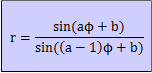Imagine two lines revolving at a constant rate round two different points (poles). The intersection of the two lines make a sectrix of Maclaurin. The name comes from the trisectrix of Maclaurin (see below), together with the sectrix quality (able to divide an angle). Parameters a and 1/a give the same curves, so we can confine ourselves to the case |a|>=1. For a=1, the curve is a line. For b=0, two curve groups can be distinguished: parameter a is an integer: the curve is called an arachnida1) or araneidan. For a=2, the curve is a circle. For a=3: we see the cubic trisectrix of Maclaurin2).Colin Maclaurin was the first to study the curve (in 1742), while looking at the ancient Greek problem of the trisection of an angle: the angle formed by points ABP is three times the angle formed by AOP for points P of the trisectrix. The area of the loop is equal to 3√3, and the distance from the origin to the point where the curve cuts the x-axis is equal to 3. Some relationships with other curves are the following: the trisectrix is a pedal of the parabola it is the polar inverse of Tschirnhausen's cubic (with the focus as center of inversion)it is the polar inverse of the hyperbola (with the nod as center of inversion) The curve is an anallagmatic curve, and also an epi spiral (a=1/3). Freeth (1819-1904) described in a paper published by the London Mathematical Society (1879) the strophoid of the trisectrix. For larger values for the parameter a we see better the spiderly character of the curve: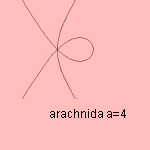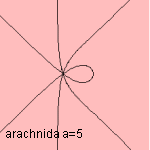A negative parameter a gives a variant on the curve, consisting of a number of (a) hyperbolic branches: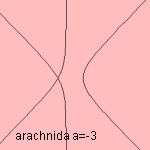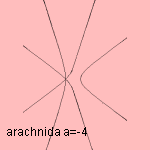parameter a is a rational number: the curve is an Plateau curve. The curve is named after the Belgian physicist Joseph Antoine Ferdinand Plateau (1801-1883). Some examples: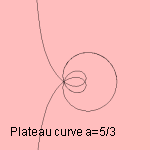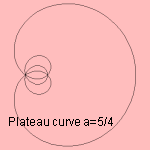For a=3/2 the curve is the (limaçon) trisectrix. When parameter a is positive, the curve consists of a number of elliptic forms. The number of elliptics is equal to the denominator of the parameter. When parameter a is negative, the curve consists of a number of hyperbolic forms. The number of hyperbolics is equal to the sum of the nominator and denominator minus 1:notes 1) From the Greek word αραχνη that means spider. 2) Cartesian equation: x3 + xy2 - 3x2 - y2 = 0, or: 2x3 + 2xy2 - 3x2 + y2 = 0.